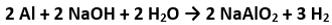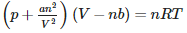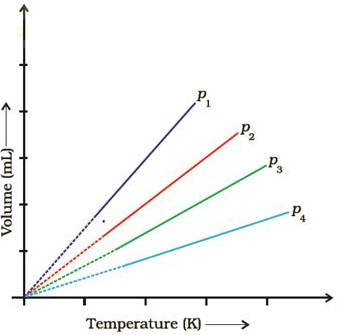Courses

# States Of Matter : Gases & Liquids - Practice Test (1)

## 25 Questions MCQ Test Additional Question Bank | States Of Matter : Gases & Liquids - Practice Test (1)

Description
This mock test of States Of Matter : Gases & Liquids - Practice Test (1) for Class 9 helps you for every Class 9 entrance exam. This contains 25 Multiple Choice Questions for Class 9 States Of Matter : Gases & Liquids - Practice Test (1) (mcq) to study with solutions a complete question bank. The solved questions answers in this States Of Matter : Gases & Liquids - Practice Test (1) quiz give you a good mix of easy questions and tough questions. Class 9 students definitely take this States Of Matter : Gases & Liquids - Practice Test (1) exercise for a better result in the exam. You can find other States Of Matter : Gases & Liquids - Practice Test (1) extra questions, long questions & short questions for Class 9 on EduRev as well by searching above.
QUESTION: 1

### The three important states of matter are

Solution:

solid,liquid and gas are the three states of matter.​

QUESTION: 2

### In the kinetic molecular theory attraction between molecules of the gas is

Solution:

KTG assume no intermolecular forces is exerting between the gas molecules.

QUESTION: 3

### The drain cleaner, Drainex contains small bits of aluminum which react with caustic soda to produce dihydrogen. What volume of dihydrogen at 20 ∘C and one bar will be released when 0.15g of aluminum reacts?

Solution:Moles of Al= 0.15/27 =0.0055 moles And 2 mole of Al produces 3 moles of H2 so 0.0055 moles will produce 3/2x0.0055 moles of H2 =0.00825 moles. Now PV=nRT where n=0.00825 , P=1 bar and T=293K.

QUESTION: 4

A person living in Shimla observed that cooking food without using pressure cooker takes more time. The reason for this observation is that at high altitude:

Solution:

As we move to higher altitude P decreases.

QUESTION: 5

Which of the following figures does not represent 1 mole of dioxygen gas at STP?

Solution:

1 mole of O2 at STP has mass 32g and volume 22.7L

QUESTION: 6

Physical behavior of the states differs greatly even though chemical behavior of the three states is identical because

Solution:

chemical properties depend on intramolecular bonding forces whereas physical properties depend on intermolecular nonbonding forces

QUESTION: 7

Real gases show deviations from ideal gas law because

Solution:

Real gases have intermolecular forces of interaction.

QUESTION: 8

What will be the pressure exerted by a mixture of 3.2 g of methane and 4.4 g of carbon dioxide contained in a 9 dmflask at 27C ?

Solution:

P=P1+P2 (Acc. To Daltons Law) And P1×9=0.2xRT And P2×9=0.1xRT where T= 300K

QUESTION: 9

Which of the following property of water can be used to explain the spherical shape of rain droplets?

Solution:

surface Tension is used.

QUESTION: 10

under which of the following two conditions, a gas deviates most from the ideal behavior?

Solution:

At High pressure and Low temperature ideal gases deviates.

QUESTION: 11

dipole-dipole forces act between molecules that have

Solution:

dipole dipole forces act between molecules that have a permanent electrical dipole moment

QUESTION: 12

Which is the correct form of van der Waals equation?

Solution:is vanderwall gas equation of real gases.

QUESTION: 13

What will be the pressure of the gaseous mixture when 0.5 L of H2 at 0.8 bar and 2.0 L of dioxygen at 0.7 bar are introduced in a 1L vessel at27C ?

Solution:

PH2x1=0.8x0.5 PO2 x 1=0.7x2 And P=PH2+PO2

QUESTION: 14

A plot of volume (V ) versus temperature (T ) for a gas at constant pressure is a straight line passing through the origin. The plots at different values of pressure are shown in Figure. Which of the following order of pressure is correct for this gas?Solution:

keep Temperature constant we know Pα1/V So as V increases P decreases.

QUESTION: 15

Which of the following changes decrease the vapour pressure of water kept in a sealed vessel?

Solution:

Adding a non volatile solute like salt decreases the vapor pressure of water

QUESTION: 16

Which of the following statements about Hydrogen bond incorrect?

Solution:

In hydrogen bonding H atom becomes partially negative and is attracted to the more positive N atom.RETAKEMORE

QUESTION: 17

behaviour of the gas becomes more ideal when

Solution:

At low P and High T condition Real gases behave as ideal.

QUESTION: 18

Density of a gas is found to be 5.46 g/dm3g/dm3 at 27 ∘C∘C at 2 bar pressure. What will be its density at STP?

Solution:

3g/dm3

QUESTION: 19

The interaction energy of London force is inversely proportional to sixth power of the distance between two interacting particles but their magnitude depends upon

Solution:

Its magnitude depends on the polarisability of interacting particles.

QUESTION: 20

Heat of vaporization is always larger than the Heat of fusion because

Solution:

Because it takes more energy to separate particles completely than just to free them from their fixed positions in the solid

QUESTION: 21

The intermolecular force primarily responsible for the condensed states of nonpolar substances is the

Solution:

The intermolecular force primarily responsible for the condensed states of nonpolar substances is the dispersion force (or London force).​

QUESTION: 22

Critical temperature (TC)of carbon dioxide is the highest temperature at which liquid carbon dioxide is observed. Above this temperature

Solution:

Tc is the highest Temp where a gas can exist in liquid state. Above this no liquid state exist.

QUESTION: 23

34.05 mL of phosphorus vapour weighs 0.0625 g at 546C and 0.1 bar pressure. What is the molar mass of phosphorus?

Solution:

PV=(0.0625/M)RT where p=0.1bar T=819.15K and V= 34.05ml

QUESTION: 24

Dipole-dipole forces act between the molecules possessing permanent dipole. Ends of dipoles possess ‘partial charges’. The partial charge is

Solution:

It is less than unit electronic charge.​

QUESTION: 25

The fraction of molecules with enough energy to escape the liquid

Solution:

The fraction of molecules with enough energy to escape the liquid is greater at the higher temperature